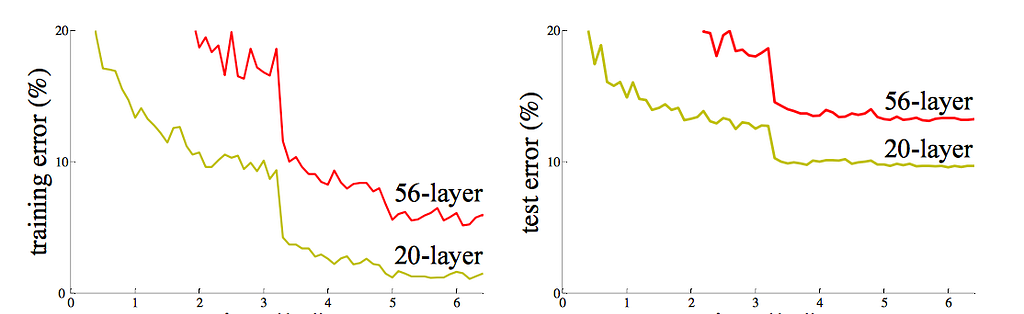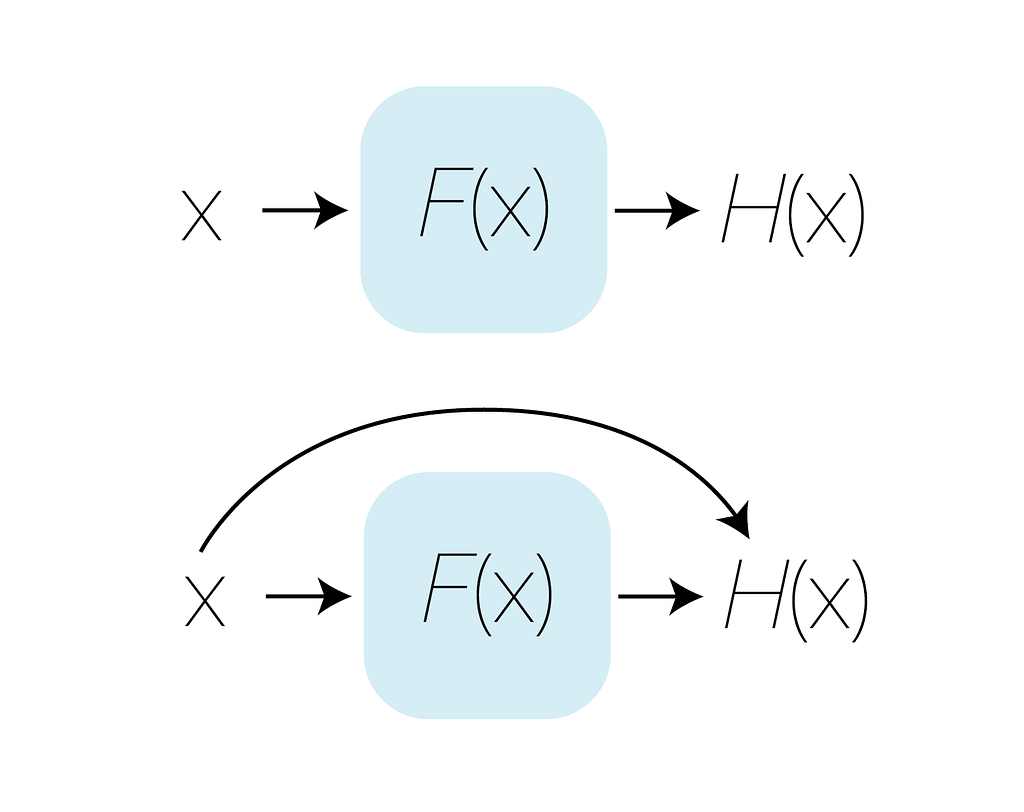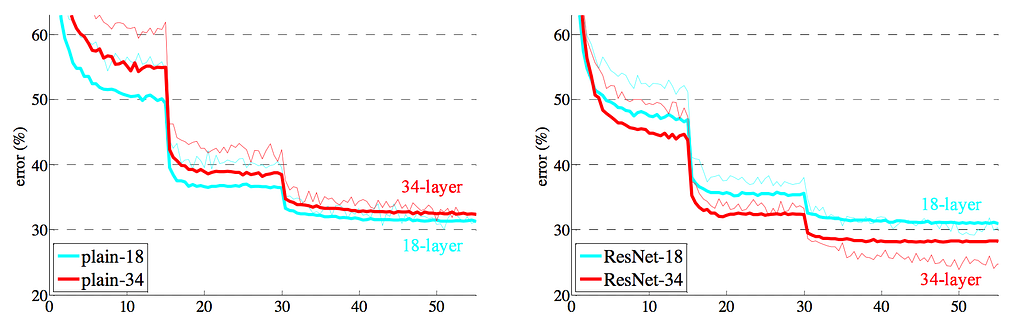# Understanding ResNet Intuitively

Every year, the ImageNet competition evaluates state-of-the-art object detection and image classification algorithms in an effort to allow researchers to compare progress and to measure the progress of the industry as a whole. In 2015, the winning algorithm utilized a novel architecture called a Deep Residual Network aka ResNet to achieve 3.7% error rate on the ImageNet dataset. To put this metric in context, the network used the year prior had an error rate almost double ResNet’s. If you’d like to read the original research paper, it can be found here. This article is meant for readers who want a guided approach to learning ResNets. If you’d like to play around with an implementation, one can be found at this Github repository

### The Problem

With the increase in popularity of Deep Learning, convolutional neural networks have become a foundational concept in image classification. In the years leading up to 2015, convolutional neural networks were part of every single architecture to win the ImageNet competition, but a significant change every year became the depth of the network itself. In 2012 AlexNet’s network was only 8 layers, in 2014, VGG-19 was 19 layers, and in 2015, Googlenet’s network was 22 layers. Based on this data, winning ImageNet seemed to be as easy as training a 100-layer network. Unfortunately that wasn’t the case as these deep networks accuracy began to decline after a certain number of layers.Figure 1: The figure above shows the results of testing the CIFAR-10 dataset on “deep” networks.

Contrary to our hypothesis, a 56-layer network performed significantly worse than a shallower network on both new data and the data it was trained on as shown in Figure 1. This is an issue known as degradation.

You are given a multi-layer neural network which maps feature x to the optimal solution H(x) with the use of a trained non-linear function F(x). Intuitively, you would think that since a deeper network has more parameters to work with, it can create more complex non-linear functions, implying the deeper network should perform at least as well as the shallower network. As seen in the experiements above, this is not the case. F(x) in a ‘plain’ network must essentially create a nonlinear function from scratch to map x to H(x). Therefore given more parameters to work with, we are essentially requiring the network to do more work than the shallower layer to accomplish the simple task of mapping a feature to itself.

### Overview

Think of any arbitrary task such as playing basketball. You might not be the best out of your friends, but you know what the best look like after watching the Golden State Warriors play all season. So the question is, how do you get from where you are to the level Stephen Curry is playing at? There are two ways of looking at your progress: “how do I be as good as Steph Curry?” and “what steps do I need to take to be as good as Steph?”. Which do you think is the better question to ask?

These two questions are asking the exact same question, but this is the same thought process that led to the key findings behind ResNets. The first question is comparable to a traditional network where you’re given a starting point and need to find a way to get to your goal or F(x)=H(x)-x. ResNet asks the second question. Given a starting point, how do you take constantly improve, taking into account your past state and your improvement at every step or H(x)=F(x)+x.

Back to the degradation problem. Let’s say x is equal to the optimal solution. In a ‘plain’ network, F(x) would still need to do the work of creating a non-linear function that maps to x. However ResNets utilize past features in it’s forward propagation. Therefore in this particular scenario, F(x) would optimize to 0 if x is already the solution. While this scenario isn’t very likely, it highlights the cause of degradation in deep networks, as well as residual learning’s approach to solving it.Figure 2: This can visually be organized by figure 2. The top image represents a traditional approach to machine learning, while the bottom image implements residual learning.

Now you may be asking, “what exactly is F(x)?”. In the implementation of a Residual Network, F(X) is no different from a “plain” convolutional neural network such as VGG, utilizing convolutional neural networks in a sequence of layers, however this small change to the architecture made a significant impact on the feasability of “deeper” networks.Figure 3: The thin curves denote training error while thicker curves show validation error. While deeper networks performed worse in plain CNNs, deep networks only improved error with ResNets.Source: Deep Learning on Medium